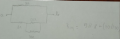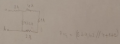# Finding the equivalent resistance of a circuit!!

#### AntonioDuarte2001

Joined Nov 1, 2020
47
I really dont understand how to know if a resistance is in parallel or in series. Can someone please give the resolution of the exercise 8 (alines "a" to "g" please) of this file.
Thanks for the help.

#### Attachments

• 215.9 KB Views: 36

#### ericgibbs

Joined Jan 29, 2010
18,212
hi A2001,
Welcome to AAC.
As this is homework, it has been moved to the Homework Forum.

Post your best attempt at answering the questions, we can then help.

Please post your questions in English.E

#### AntonioDuarte2001

Joined Nov 1, 2020
47
Thank you for replying. I am trying to find the equivalent resistance of 5 different circuits.
I sent a photo of my resolution efforts.
I tried to simplify some circuits before calculating the equivalent resistance.
They are probably wrong, i appreciate all the help and explanations that you guys can give me!

#### MrChips

Joined Oct 2, 2009
29,794
Resistors in parallel are connected to the same two nodes. The voltage across each resistor is therefore the same.
Two resistors in series have the same current flowing through them. The two share a common node with no other resistor.

#### WBahn

Joined Mar 31, 2012
29,476
I really dont understand how to know if a resistance is in parallel or in series. Can someone please give the resolution of the exercise 8 (alines "a" to "g" please) of this file.
Thanks for the help.
You are being very careless with your order of operations and this is going to bring you to grief.

For instance, inYou say:

Req = 5 Ω || 8 Ω + (20 Ω || 30 Ω)

and what you appear to mean is:

Req = 5 Ω || [8 Ω + (20 Ω || 30 Ω)]

which requires that a series combination take precedence of parallel combination.

But then inYou say:

Req = ( 8 Ω + 4.62 Ω || 4Ω + 1 Ω + 2 Ω )

Using what you previously established, this would have to be read as

Req = ( (8 Ω + 4.62 Ω) || (4Ω + 1 Ω + 2 Ω) )

but what you appear to mean is:

Req = ( 8 Ω + { 4.62 Ω || [4Ω + 1 Ω + 2 Ω] } )

and in this case you require that series combination take precedence over parallel combination on the right portion but that parallel combination take precedence over series combination on the left portion.

You can't have it both ways.

Do yourself a HUGE favor and ALWAYS make how things are going to combine EXPLICIT -- you WILL save yourself a LOT of problems.

#### AntonioDuarte2001

Joined Nov 1, 2020
47
I understand what you re saying, but i still can´t find the right result in this exercises...

#### ericgibbs

Joined Jan 29, 2010
18,212
hi A2001,
I have the PDF which circuit from that PDF are you have a problem with.?
E

#### AntonioDuarte2001

Joined Nov 1, 2020
47
hi A2001,
I have the PDF which circuit from that PDF are you have a problem with.?
E
The circuits 8c, 8d, 8e, 8f and 8g. Thank you.

#### AntonioDuarte2001

Joined Nov 1, 2020
47

#### WBahn

Joined Mar 31, 2012
29,476
I understand what you re saying, but i still can´t find the right result in this exercises...
This is why we need to see your work. We are not psychics. We cannot tell you what you did wrong unless you show us what you did. Sometimes we can make an educated guess about what you did wrong from knowing the wrong answer that you got, but you won't even tell us that much.

Let's focus on your first one, the one where you said that the equivalent resistance is given by

Req = 5 Ω || 8 Ω + (20 Ω || 30 Ω)

What are the steps you took to evaluate this and what answer did you end up with?

Did you, by chance, end up with an answer of 15.1 Ω?

Last edited:

#### ericgibbs

Joined Jan 29, 2010
18,212
hi 2001,
OK, Lets name the 12 Ohm as R5
So what is the equivalent resistance value of R2 and R5 in series.?
E

#### AntonioDuarte2001

Joined Nov 1, 2020
47
hi 2001,
OK, Lets name the 12 Ohm as R5
So what is the equivalent resistance value of R2 and R5 in series.?
E
20 ohm.

#### ericgibbs

Joined Jan 29, 2010
18,212
hi
OK, Lets name the 20 Ohm as R6.
What is the value of the equivalent resistance of R1and R6 in parallel. ?

E

#### AntonioDuarte2001

Joined Nov 1, 2020
47
hi
OK, Lets name the 20 Ohm as R6.
What is the value of the equivalent resistance of R1and R6 in parallel. ?

E
5.71 ohm.

#### ericgibbs

Joined Jan 29, 2010
18,212
hi,
No, redo it.!
How can a 5 Ohm and a 20 Ohm give a Decimal value.????

Requiv = (5*20)/(5+20) ===== ?
E

#### AntonioDuarte2001

Joined Nov 1, 2020
47
hi,
No, redo it.!
How can a 5 Ohm and a 20 Ohm give a Decimal value.????

Requiv = (5*20)/(5+20) ===== ?
E
My mistake. It is 4ohm. But in the solutions the result is "5.82 ohm".

#### ericgibbs

Joined Jan 29, 2010
18,212
hi,

5*20 = 100 Ohms
5+20 = 25 Ohms

So 100/25 =4 Ohms

Where is the '"5.82" in the Solutions.?

E

#### AntonioDuarte2001

Joined Nov 1, 2020
47
hi,
No,,try again..5*20 = 100 Ohms
5+20 = 25 Ohms

So 100/25 =====.?

E
Like i said the result is 4ohm..

#### ericgibbs

Joined Jan 29, 2010
18,212
hi,
You are correct 4 Ohms.So now to complete this circuit, post the individual equations for each step we have done, then end with a complete equation for the circuit, so that we can see that you understand the importance of the location of the brackets in the equations.

This is one of the points that WBahn was making